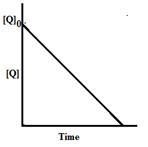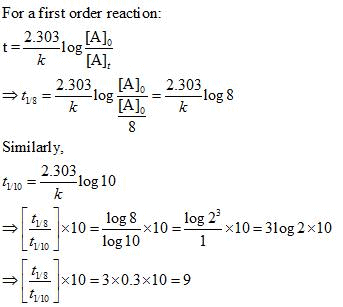Courses

# Notes | EduRev

## JEE : Notes | EduRev

The document Notes | EduRev is a part of the JEE Course Mock Test Series for JEE Main & Advanced 2021.
All you need of JEE at this link: JEE

Question 1: The rate of a reaction doubles when its temperature changes from 300K to 310K. Activation energy of such a reaction will be: (R = 8.314 JK–1mol–1and log 2 = 0.301)    (IIT JEE-2013)
(a) 48.6 kJ mol–1
(b) 58.5 kJ mol–1
(c) 60.5 kJ mol–1
(d) 53.6 kJ mol–1
Answer: d
Solution: The question is based on the effect of temperature change on rate of reaction and involves the concept of activation energy. So, Arrhenius equation should be used for solving this problem.
Using Arrhenius Equation
ln(k2/k1) = (Ea/R)(1/T1-1/T2)
We get,
2.303 log2 = -( Ea/8.314)(1/300 -1/310)
Ea = 5359.59 Jmol-1 = 53.6 kJ mol-1
Hence, the correct option is D.

Question 2: In the reaction P + Q → R + S, the time taken for 75% reaction of P is twice the time taken for 50% reaction of P. The concentration of Q varies with reaction time as shown in the figure. The overall order of the reaction is    (IIT JEE -2013)(a) 2
(b) 3
(c) 0
(d) 1
Answer: d
Solution:
Time required for 75% reaction of P is twice the time taken for 50% reaction, this indicates that the half life of the reaction is independent of the initial concentration and remains constant throughout the reaction.
For first order kinetics, after first half life the concentration of P decreases up to 50 %.
After 2nd half life the concentration of P once again decreases to its half value and now it reduced up to 25% of the initial concentration.
From the graph it is clear that reaction is zero order with respect to Q.
R =k1[P]1[Q]0
Overall order of the reaction = 1+0 =1.
Hence, the correct option is D.

Question 3: An organic compound undergoes first-order decomposition. The time taken for its decomposition to 1/8 and 1/10 of its initial concentration are t1/8 and t1/10 respectively. What is the value of [t1/8 ] / [t1/10 ] × 10 ?  (Take log2 = 0.3)    (IIT JEE -2012)
Solution:Offer running on EduRev: Apply code STAYHOME200 to get INR 200 off on our premium plan EduRev Infinity!

## Mock Test Series for JEE Main & Advanced 2021

6 videos|259 docs|164 tests

,

,

,

,

,

,

,

,

,

,

,

,

,

,

,

,

,

,

,

,

,

;